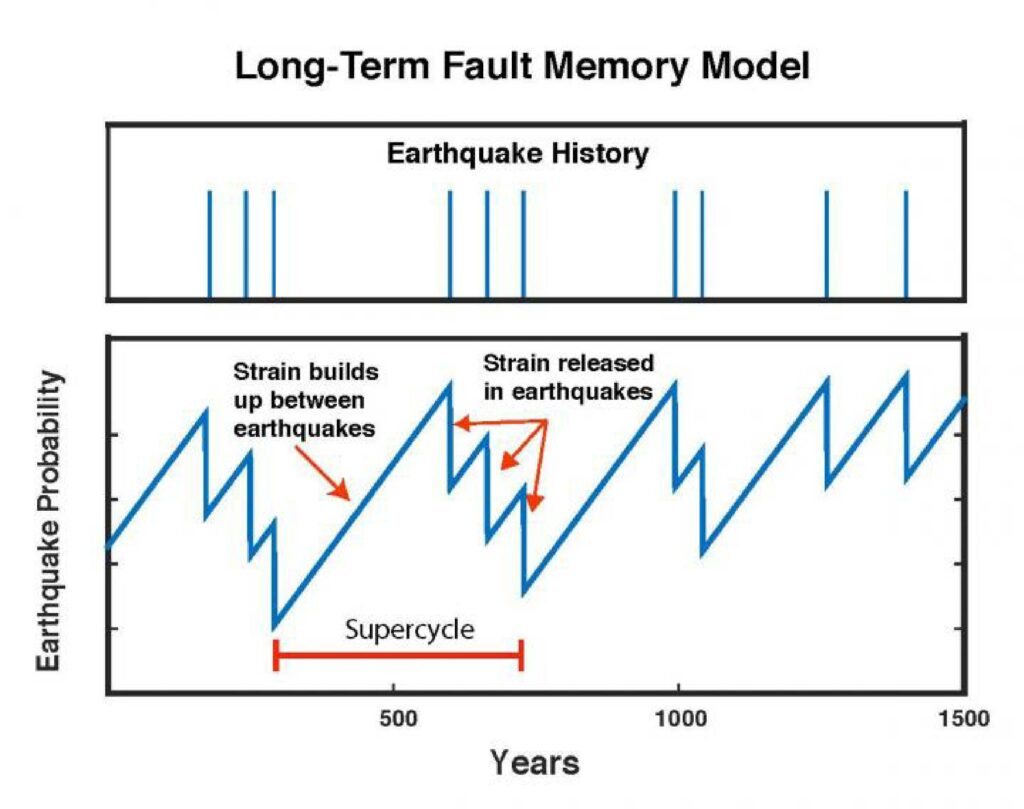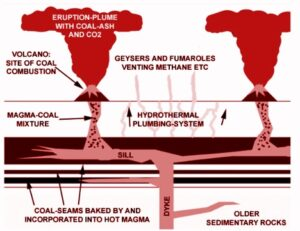January 28, 2023

# Assignment EQ super cycle1. Guess the Length of super cycle (time interval between the two successive M-9 earthquakes) assuming that the earthquake fully releases the stress associated with the Pacific plate subduction.

Ans:

From the New model explaining the coexistence of frequent M-8 and infrequent M9 earthquakes we find that, to complete one Super cycle it requires approximately 571 years because from the past record we know that, in 869 BC, 1611BC? and in 2011 BC there are three successive large earthquake occurred and completing three super cycles by releasing all strain of strong coupling.

According to this new model, when pacific plate subducts beneath the North American continental plate all strain is not released by small asperities but accumulated for a long time. Then by releasing entire strain a large infrequent earthquake (M9) is occurred and complete a super cycle.

So, from the new model;

{(1611-869)+(2011-1611)}/2

=571 year

From the above conclusion we can guess the length of super cycle is 571 years.

2. Guess the amount of coseismic slip associated with usual M-8 earthquake, assuming that seismic coupling coefficient is 0.8 and recurrence time interval is 100 years.

Ans;

We know, coupling  coefficient , x=D/vT0

>  80%=D/8*100

Therefore D=640 cm=64 meter (ans).

Where,

X= coupling  coefficient= 0.8=80%

D=coseismic slip

V=motion of plate =8 cm/year

T0=Recurrence interval=100 years

So, we can guess the amount of coseismic slip (D) is 64 m.

#### You may have missed#### Causes of Permian Mass extinction